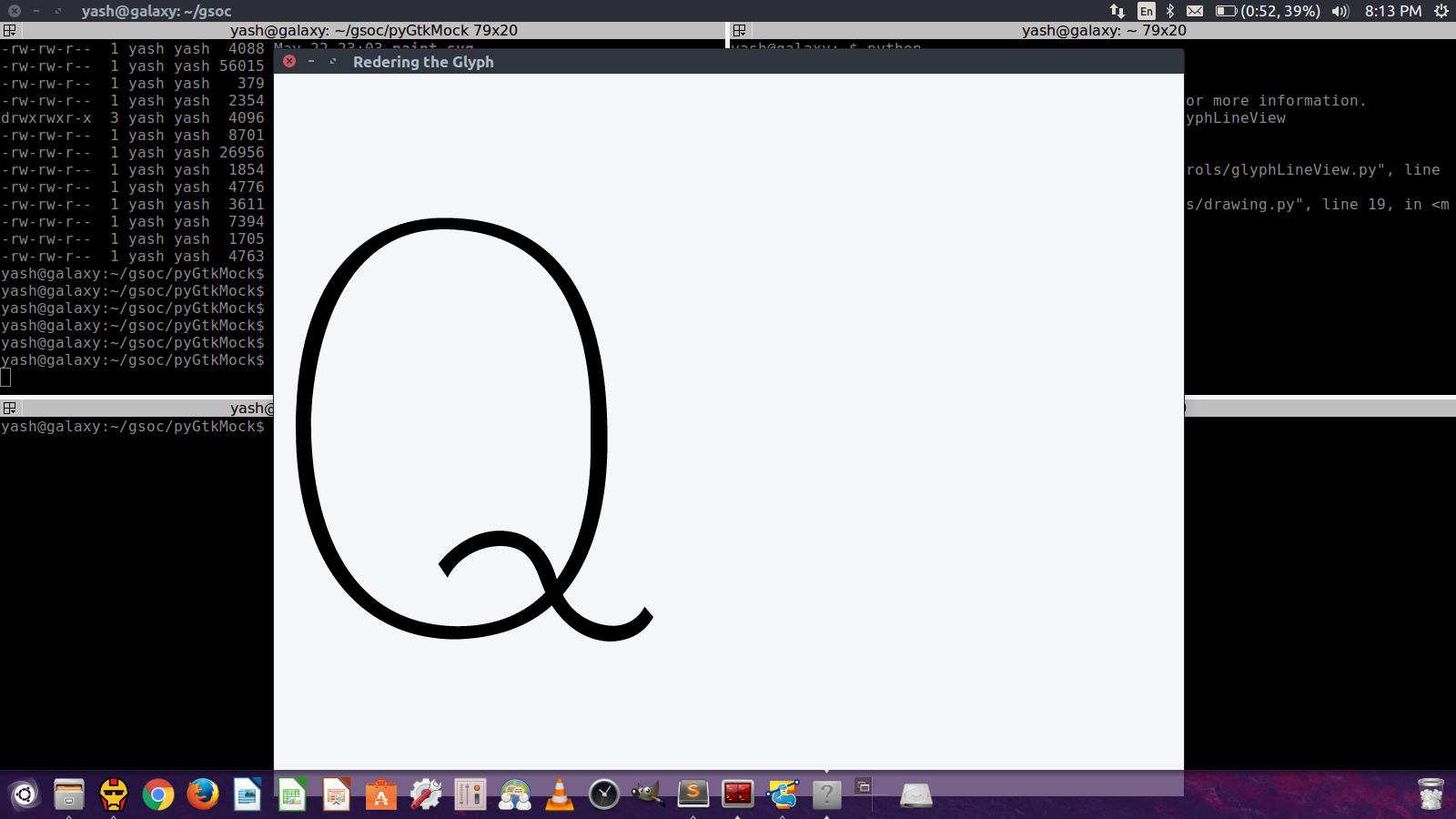Check The GitHub Repository

# Drawing UFO GLIF outlines with Cairo

2016-05-23

Yash has already got glyph rendering going!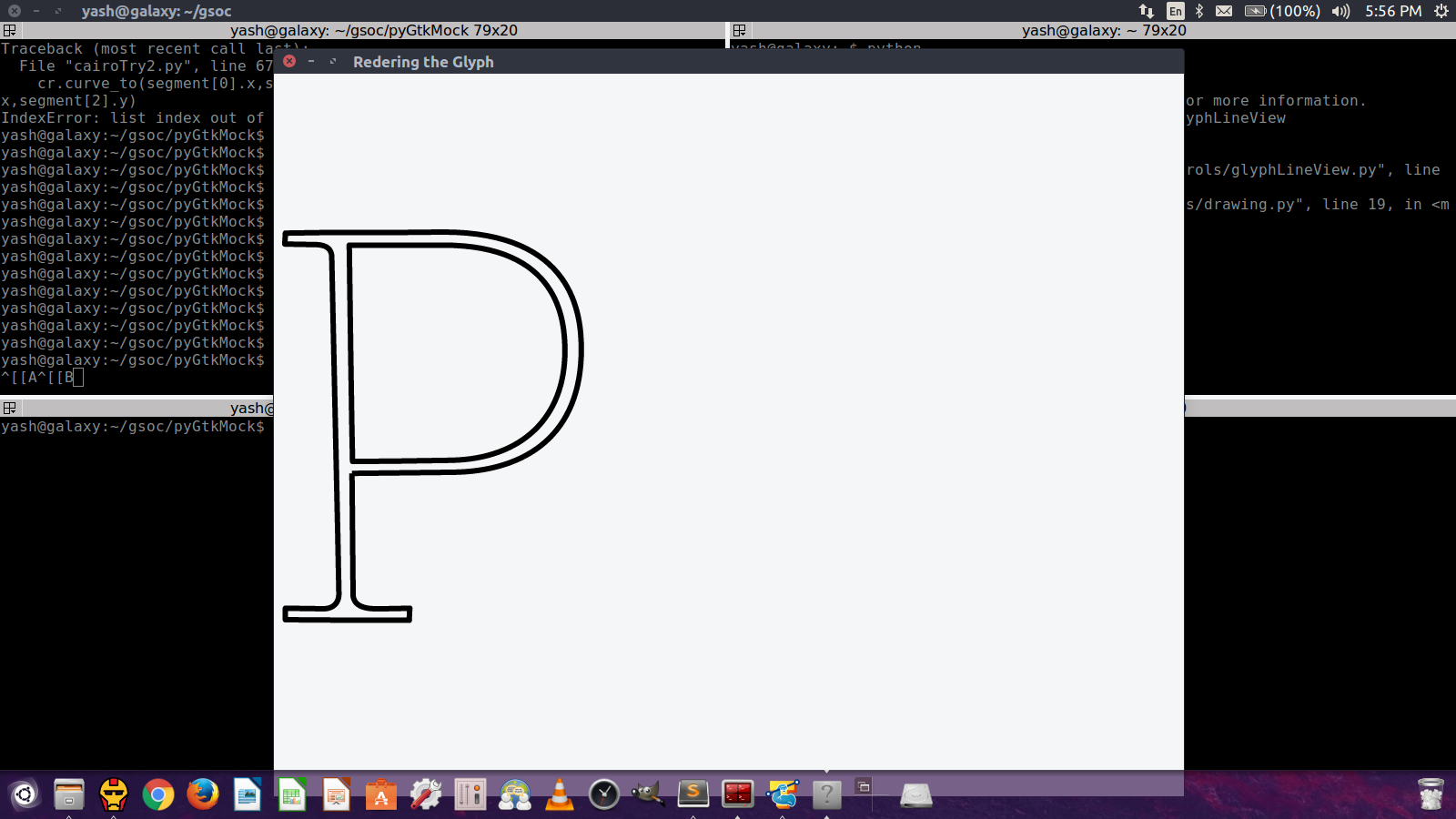However, when filling the glyph, it isn’t rendering the “counter” or white-shape: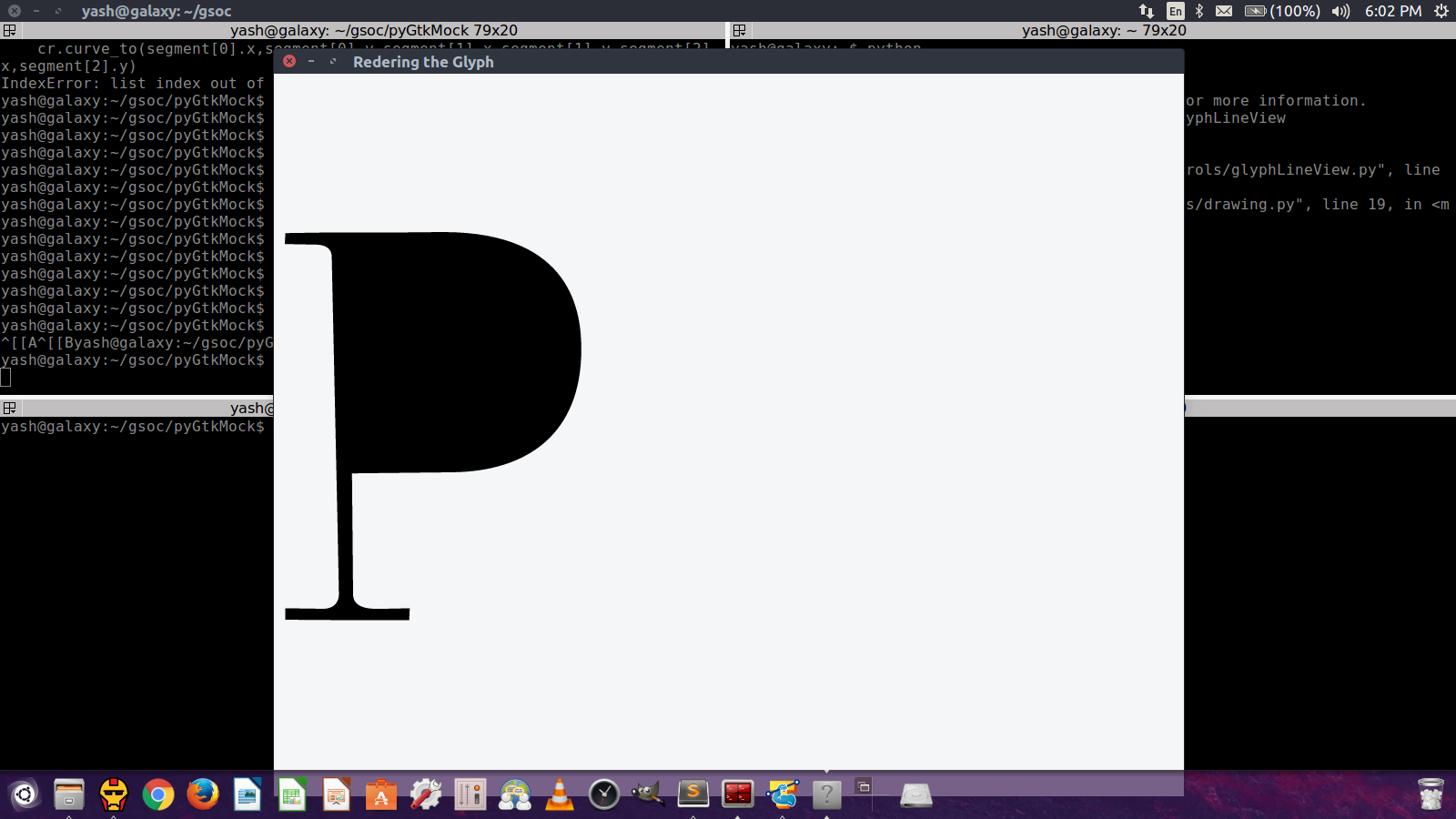The reason is that the default “fill rule” is using the “winding directions” of the contours. The pycairo documentation explains,

Whether or not a point is included in the fill is determined by taking a ray from that point to infinity and looking at intersections with the path. The ray can be in any direction, as long as it doesn’t pass through the end point of a segment or have a tricky intersection such as intersecting tangent to the path. (Note that filling is not actually implemented in this way. This is just a description of the rule that is applied.)

The default fill rule is `FILL_RULE_WINDING`:

``````cairo.FILL_RULE_WINDING
If the path crosses the ray from left-to-right, counts +1.
If the path crosses the ray from right to left, counts -1.
(Left and right are determined from the perspective of
looking along the ray from the starting point.)
If the total count is non-zero, the point will be filled.
``````

But there is another fill rule:

``````cairo.FILL_RULE_EVEN_ODD
Counts the total number of intersections, without regard
to the orientation of the contour.
If the total number of intersections is odd, the point
will be filled.
``````

https://cairographics.org/documentation/pycairo/2/reference/constants.html#constants-fill-rule

While `FILL_RULE_WINDING` is the rule used for PostScript, a simplified editor for kids should use `FILL_RULE_EVEN_ODD` and a ‘correct directions’ method should be auto-applied, so that kids don’t have to think about winding directions.

Here is a simple demonstration, derived from the example in https://www.cairographics.org/pycairo/tutorial/:

``````#!/usr/bin/env python

import math
import cairo

WIDTH, HEIGHT = 256, 256

def draw(output, evenodd=False):
surface = cairo.ImageSurface (cairo.FORMAT_ARGB32, WIDTH, HEIGHT)
ctx = cairo.Context (surface)
ctx.scale (WIDTH, HEIGHT) # Normalizing the canvas
if evenodd:
ctx.set_fill_rule(cairo.FILL_RULE_EVEN_ODD)

pat = cairo.LinearGradient (0.0, 0.0, 0.0, 1.0)
pat.add_color_stop_rgba (1, 0.7, 0, 0, 0.5) # First stop, 50% opacity
pat.add_color_stop_rgba (0, 0.9, 0.7, 0.2, 1) # Last stop, 100% opacity

ctx.rectangle (0, 0, 1, 1) # Rectangle(x0, y0, x1, y1)
ctx.set_source (pat)
ctx.fill ()

ctx.translate (0.1, 0.1) # Changing the current transformation matrix
ctx.set_source_rgb (0.3, 0.2, 0.5) # Solid color
ctx.set_line_width (0.02)

ctx.move_to (0, 0)
ctx.line_to (0.5, 0.1) # Line to (x,y)
ctx.curve_to (0.5, 0.2, 0.5, 0.4, 0.2, 0.8) # Curve(x1, y1, x2, y2, x3, y3)
ctx.close_path ()

ctx.translate (0.15, 0.15) # Changing the current transformation matrix
ctx.set_source_rgb (0.5, 0.5, 0.5) # Solid color
ctx.set_line_width (0.2)
ctx.move_to (0, 0)
ctx.line_to (0.3, 0.1) # Line to (x,y)
ctx.curve_to (0.3, 0.2, 0.1, 0.1, 0.1, 0.25) # Curve(x1, y1, x2, y2, x3, y3)
ctx.close_path ()
ctx.fill ()
ctx.stroke ()

surface.write_to_png(output) # Output to PNG

draw("2016-05-23-fill-example-1.png")
draw("2016-05-23-fill-example-2.png", evenodd=True)
``````

Here is 2016-05-23-fill-example-1.png: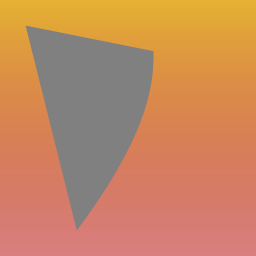And here is 2016-05-23-fill-example-2.png with the “evenodd” fill rule set: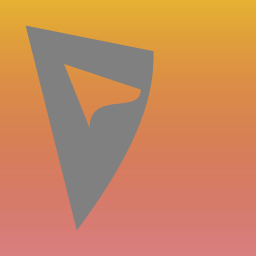And with d959d38 Yash has cracked it!